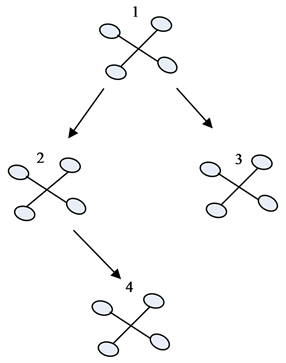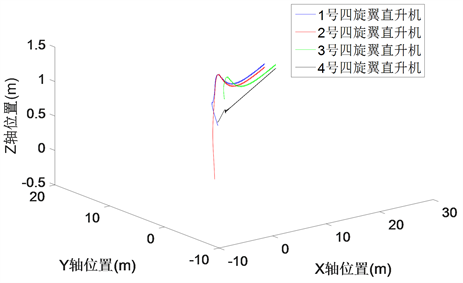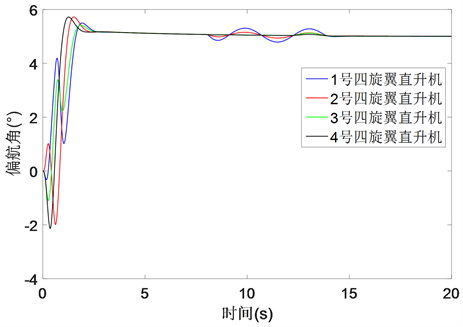#### 期刊菜单

Fault Cooperative Tolerant Control for Multiple Quadrotor Systems with Sensor Faults
DOI: 10.12677/JAST.2021.94014, PDF, HTML, XML, 下载: 140  浏览: 243  科研立项经费支持

Abstract: Due to the local communication among individuals in multiple quadrotor systems, once some quadrotors in the formation have sensor faults, the faulty quadrotors and the ones which can receive the information from them may deviate from the desired path such that the predesigned formation shape cannot be maintained. Thus, a distributed control scheme based on the dynamic surface control is proposed for multiple quadrotor systems with unknown senor faults to maintain a leader-follower formation. Moreover, the leader can always follow the external reference signals under the designed controllers. By doing simulation studies on Qball-X4 quadrotors, the results show the effectiveness of the proposed method.

1. 引言Figure 1. Schematic diagram for the topology of the considered leader- follower formation

2. 基础知识

$\mathcal{G}\triangleq \left(\mathcal{V},\mathcal{E},\mathcal{A}\right)$，它由n个节点和m条有向弧组成，所有节点和有向弧分别组成节点集 $\mathcal{V}=\left\{1,2,\cdots ,n\right\}$ 和有向弧集 $\mathcal{E}=\left\{{\mathcal{E}}^{1},\cdots ,{\mathcal{E}}^{m}\right\}$。其中每条有向弧都代表着2个节点之间的有向连接，例如有向弧 $\left(i,j\right)\in \mathcal{E}$ 表明节

${\mathcal{N}}_{i}\triangleq \left\{j\in \mathcal{V}|\left(j,i\right)\in \mathcal{E}\right\}$

${R}_{i}\triangleq \left\{j\in \mathcal{V}|\left(i,j\right)\in \mathcal{E}\right\}$

${\mathcal{A}}_{ij}=\left\{\begin{array}{l}1,\text{\hspace{0.17em}}\text{\hspace{0.17em}}\text{ }\text{ }若\left(j,i\right)\in \mathcal{E}\hfill \\ 0,\text{\hspace{0.17em}}\text{\hspace{0.17em}}否则\hfill \end{array}$

$L\triangleq D-\mathcal{A}$

3. 问题描述

1) 每个节点i ( $i\in \mathcal{V}$ )需跟随其父节点，而主节点则需跟踪参考信号；

2) 每个节点i ( $i\in \mathcal{V}$ )需与其邻集中的每个节点j ( $j\in {\mathcal{N}}_{i}$ )保持预设的相对距离，该相对距离分解到惯性坐标系后，定义为 ${d}_{ij}\triangleq {\left[{d}_{ijx},{d}_{ijy},{d}_{ijz}\right]}^{\text{T}}$，如图2所示。Figure 2. The relative distance between node i and node j in the inertial frame

$\left\{\begin{array}{l}{\stackrel{¨}{x}}_{i}={u}_{i1}\left(\mathrm{cos}{\varphi }_{i}\mathrm{sin}{\theta }_{i}cos{\psi }_{i}+\mathrm{sin}{\varphi }_{i}\mathrm{sin}{\psi }_{i}\right)\\ {\stackrel{¨}{y}}_{i}={u}_{i1}\left(\mathrm{cos}{\varphi }_{i}\mathrm{sin}{\theta }_{i}\mathrm{sin}{\psi }_{i}-\mathrm{sin}{\varphi }_{i}\mathrm{cos}{\psi }_{i}\right)\\ {\stackrel{¨}{z}}_{i}={u}_{i1}\mathrm{cos}{\varphi }_{i}\mathrm{cos}{\theta }_{i}-g\\ {\stackrel{¨}{\theta }}_{i}={u}_{i2}+\frac{{J}_{\varphi }-{J}_{\psi }}{{J}_{\theta }}{\stackrel{˙}{\psi }}_{i}{\stackrel{˙}{\varphi }}_{i}\\ {\stackrel{¨}{\varphi }}_{i}={u}_{i3}+\frac{{J}_{\psi }-{J}_{\theta }}{{J}_{\varphi }}{\stackrel{˙}{\psi }}_{i}{\stackrel{˙}{\theta }}_{i}\\ {\stackrel{¨}{\psi }}_{i}={u}_{i4}+\frac{{J}_{\theta }-{J}_{\varphi }}{{J}_{\psi }}{\stackrel{˙}{\varphi }}_{i}{\stackrel{˙}{\theta }}_{i}\end{array}$ (1)

$\left\{\begin{array}{l}{\stackrel{˙}{w}}_{i1}={w}_{i2}\\ {\stackrel{˙}{w}}_{i2}={u}_{i1}{g}_{1}\left({w}_{i5}\right)\tau \left({w}_{i3}\right)\\ {\stackrel{˙}{w}}_{i3}={w}_{i4}\\ {\stackrel{˙}{w}}_{i4}={v}_{i1}+{f}_{i1}\end{array}$ (2)

$\left\{\begin{array}{l}{\stackrel{˙}{w}}_{i5}={w}_{i6}\\ {\stackrel{˙}{w}}_{i6}={g}_{2}\left({w}_{i3}\right){v}_{i2}+{f}_{i2}\end{array}$ (3)

${g}_{1}=\frac{1}{m}\left[\begin{array}{cc}\mathrm{sin}{\psi }_{i}& \mathrm{cos}{\psi }_{i}\\ -\mathrm{cos}{\psi }_{i}& \mathrm{sin}{\psi }_{i}\end{array}\right],\text{\hspace{0.17em}}{g}_{2}=\left[\begin{array}{cc}\frac{\mathrm{cos}{\varphi }_{i}\mathrm{cos}{\theta }_{i}}{m}& 0\\ 0& 1\end{array}\right]$

${f}_{i1}=\left[\begin{array}{c}\frac{{J}_{\varphi }-{J}_{\psi }}{{J}_{\theta }}{\stackrel{˙}{\psi }}_{i}{\stackrel{˙}{\varphi }}_{i}\\ \frac{{J}_{\psi }-{J}_{\theta }}{{J}_{\varphi }}{\stackrel{˙}{\psi }}_{i}{\stackrel{˙}{\theta }}_{i}\end{array}\right],\text{\hspace{0.17em}}{f}_{i2}=\left[\begin{array}{c}-g\\ \frac{{J}_{\theta }-{J}_{\varphi }}{{J}_{\psi }}{\stackrel{˙}{\varphi }}_{i}{\stackrel{˙}{\theta }}_{i}\end{array}\right]$

$\tau \left({w}_{i3}\right)=\left[\begin{array}{c}\mathrm{sin}{\varphi }_{i}\\ \mathrm{cos}{\varphi }_{i}\mathrm{sin}{\theta }_{i}\end{array}\right]$

$\left\{\begin{array}{l}{y}_{oi_1}={x}_{i1}\\ {y}_{oi_2}={x}_{i2}\end{array}$

$r\triangleq {\left[{x}_{r},{y}_{r},{z}_{r},{\psi }_{r}\right]}^{\text{T}}$，其余所有僚机都无法直接获知参考信号r的信息，因此如果系统中缺少协同控制，则

$\left\{\begin{array}{l}{y}_{oi_1}={x}_{i1}\\ {y}_{oi_2}={x}_{i2}+{f}_{si}\end{array}$ (4)

${b}_{i}\left(t\right)=\left\{\begin{array}{l}{b}_{i},\text{\hspace{0.17em}}\text{\hspace{0.17em}}{\stackrel{˙}{b}}_{i}\left(t\right)\equiv 0,\text{\hspace{0.17em}}\text{\hspace{0.17em}}偏移故障\hfill \\ {b}_{i}\left(t\right),\text{\hspace{0.17em}}\text{\hspace{0.17em}}|{b}_{i}\left(t\right)|={\lambda }_{i}t,\text{\hspace{0.17em}}\text{\hspace{0.17em}}漂移故障\hfill \\ {b}_{i}\left(t\right),\text{\hspace{0.17em}}\text{\hspace{0.17em}}|{b}_{i}\left(t\right)|<{\stackrel{¯}{b}}_{i},\text{\hspace{0.17em}}{\stackrel{˙}{b}}_{i}\left(t\right)\to 0,\text{\hspace{0.17em}}\text{\hspace{0.17em}}精度损失\hfill \end{array}$

$\left\{\begin{array}{l}{\stackrel{˙}{w}}_{i1}={w}_{i2}-{f}_{si1}\\ {\stackrel{˙}{w}}_{i2}={u}_{i1}{g}_{1}\left({w}_{i5}\right)\tau \left({w}_{i3}\right)\\ {\stackrel{˙}{w}}_{i3}={w}_{i4}-{f}_{si2}\\ {\stackrel{˙}{w}}_{i4}={v}_{i1}+{f}_{i1}+\delta {f}_{i1}\end{array}$ (5)

$\left\{\begin{array}{l}{\stackrel{˙}{w}}_{i5}={w}_{i6}-{f}_{si3}\\ {\stackrel{˙}{w}}_{i6}={g}_{2}\left({w}_{i3}\right){v}_{i2}+{f}_{i2}+\delta {f}_{i2}\end{array}$ (6)

${\left[{x}_{1},{y}_{1},{z}_{1},{\psi }_{1}\right]}^{\text{T}}\to r$${\left[{x}_{i}-{x}_{j},{y}_{i}-{y}_{j},{z}_{i}-{z}_{j}\right]}^{\text{T}}\to {d}_{ij}$${\psi }_{i}-{\psi }_{j}\to 0$，其中 $j\in {\mathcal{N}}_{i}$

4. 协同容错控制设计

${\sigma }_{i1}\triangleq {w}_{i2}-{b}_{i}{w}_{r2}+{b}_{i}\left({w}_{i1}-{w}_{r1}\right)+\underset{j\in {\mathcal{N}}_{i}}{\sum }{a}_{ij}\left({w}_{i1}-{w}_{j1}-{d}_{ijxy}\right)$ (7)

${\sigma }_{i2}\triangleq {w}_{i3}-{w}_{i3d}$ (8)

${\sigma }_{i3}\triangleq {w}_{i4}-{w}_{i4d}$ (9)

${\sigma }_{i4}\triangleq {w}_{i6}-{b}_{i}{w}_{r6}+{b}_{i}\left({w}_{i5}-{w}_{r5}\right)+\underset{j\in {\mathcal{N}}_{i}}{\sum }{a}_{ij}\left({w}_{i5}-{w}_{j5}-{h}_{ij}\right)$ (10)

${b}_{i}=\left\{\begin{array}{l}1,\text{\hspace{0.17em}}\text{\hspace{0.17em}}i=1,\text{\hspace{0.17em}}\text{\hspace{0.17em}}即\text{\hspace{0.17em}}i\text{\hspace{0.17em}}为主机\hfill \\ 0,\text{\hspace{0.17em}}\text{\hspace{0.17em}}i\in \mathcal{V}-\left\{1\right\},\text{\hspace{0.17em}}\text{\hspace{0.17em}}即\text{\hspace{0.17em}}i\text{\hspace{0.17em}}为僚机\hfill \end{array}$ (11)

$\begin{array}{c}{\stackrel{˙}{w}}_{i3d}=\frac{{J}^{-1}\left({w}_{i3d}\right)}{{\epsilon }_{1}}\left\{\frac{{g}_{1}^{-1}}{{u}_{i1}}×\left[-{k}_{1}{\sigma }_{i1}-{b}_{i}\left({w}_{i2}-{\stackrel{^}{f}}_{si1}-{w}_{r2}\right)-\underset{j\in {\mathcal{N}}_{i}}{\sum }{a}_{ij}\left({w}_{i2}-{\stackrel{^}{f}}_{si1}-{w}_{j2}\right)\\ \text{\hspace{0.17em}}+{b}_{i}{\stackrel{˙}{w}}_{r2}-{\epsilon }_{1}\frac{\text{d}{u}_{i1}{g}_{1}}{\text{d}t}\tau \left({w}_{i3d}\right)\right]-\tau \left({w}_{i3d}\right)\right\}\end{array}$ (12)

${\stackrel{˙}{w}}_{i4d}=\frac{1}{{\epsilon }_{2}}\left(-{k}_{2}{\sigma }_{i3}+{\stackrel{˙}{w}}_{i3d}+{\stackrel{^}{f}}_{si2}-{w}_{i4d}\right)$ (13)

${v}_{i1},\text{\hspace{0.17em}}{v}_{i2}$ 设计成如下形式：

${v}_{i1}=-{k}_{3}{\sigma }_{i3}+{\stackrel{˙}{w}}_{i4d}-{f}_{i1}-\delta {\stackrel{^}{f}}_{i1}$ (14)

${v}_{i2}={g}_{2}^{-1}\left[-{k}_{4}{\sigma }_{i4}-{f}_{i2}-\delta {\stackrel{^}{f}}_{i2}-\underset{j\in {\mathcal{N}}_{i}}{\sum }{a}_{ij}\left({w}_{i6}-{\stackrel{^}{f}}_{si3}-{w}_{j6}\right)-{b}_{i}\left({w}_{i6}-{\stackrel{^}{f}}_{si3}-{w}_{r6}\right)+{b}_{i}{\stackrel{˙}{w}}_{r6}\right]$ (15)

${\stackrel{˙}{\stackrel{^}{f}}}_{si1}=-{k}_{1}\left[\left(\underset{j\in {\mathcal{N}}_{i}}{\sum }{a}_{ij}+{b}_{i}\right){e}_{i1}-\underset{k\in ch\left(i\right)}{\sum }{a}_{ki}{e}_{k1}\right]+{\beta }_{1}{\stackrel{˜}{f}}_{si1}$ (16)

${\stackrel{˙}{\stackrel{^}{f}}}_{si2}=-{\sigma }_{i2}+{\beta }_{2}{\stackrel{˜}{f}}_{si2}$ (17)

${\stackrel{˙}{\stackrel{^}{f}}}_{si3}=-\left(\underset{j\in {\mathcal{N}}_{i}}{\sum }{a}_{ij}+{b}_{i}\right){\sigma }_{i4}+\underset{k\in ch\left(i\right)}{\sum }{a}_{ki}{\sigma }_{k4}+{\beta }_{3}{\stackrel{˜}{f}}_{si3}$ (18)

$\delta {\stackrel{˙}{\overline{)\stackrel{^}{f}}}}_{i1}={\sigma }_{i3}+{\beta }_{4}\delta {\stackrel{˜}{f}}_{i1}$ (19)

$\delta {\stackrel{˙}{\overline{)\stackrel{^}{f}}}}_{i2}={\sigma }_{i4}+{\beta }_{5}\delta {\stackrel{˜}{f}}_{i2}$ (20)

$\begin{array}{c}{\stackrel{˙}{w}}_{j3d}=\frac{{J}^{-1}\left({w}_{j3d}\right)}{{\epsilon }_{1}}\left\{\frac{{g}_{1}^{-1}}{{u}_{j1}}×\left[-{k}_{1}{\sigma }_{j1}-{b}_{j}\left({w}_{j2}-{w}_{r2}\right)-\underset{k\in {\mathcal{N}}_{j}}{\sum }{a}_{jk}\left({w}_{j2}-{w}_{k2}\right)\\ \text{\hspace{0.17em}}+{b}_{j}{\stackrel{˙}{w}}_{r2}-{a}_{ji}{\stackrel{^}{f}}_{si1}-{\epsilon }_{1}\frac{\text{d}{u}_{j1}{g}_{1}}{\text{d}t}\tau \left({w}_{j3d}\right)\right]-\tau \left({w}_{j3d}\right)\right\}\end{array}$ (21)

${\stackrel{˙}{w}}_{j4d}=\frac{1}{{\epsilon }_{2}}\left(-{k}_{2}{\sigma }_{j3}+{\stackrel{˙}{w}}_{j3d}-{w}_{j4d}\right)$ (22)

${\stackrel{˙}{v}}_{j1}=-{k}_{3}{\sigma }_{j3}+{\stackrel{˙}{w}}_{j4d}-{f}_{j1}$ (23)

${v}_{j2}={g}_{2}^{-1}\left[-{k}_{4}{\sigma }_{j4}-{f}_{j2}-\underset{k\in {\mathcal{N}}_{j}}{\sum }{a}_{jk}\left({w}_{j6}-{w}_{j6}\right)-{a}_{ji}{\stackrel{^}{f}}_{si3}-{b}_{j}\left({w}_{j6}-{w}_{r6}\right)+{b}_{j}{\stackrel{˙}{w}}_{r6}\right]$ (24)

$\begin{array}{c}{\stackrel{˙}{w}}_{k3d}=\frac{{J}^{-1}\left({w}_{k3d}\right)}{{\epsilon }_{1}}\left\{\frac{{g}_{1}^{-1}}{{u}_{k1}}×\left[-{k}_{1}{\sigma }_{k1}-{b}_{k}\left({w}_{k2}-{w}_{r2}\right)-\underset{i\in {\mathcal{N}}_{k}}{\sum }{a}_{ki}\left({w}_{k2}-{w}_{i2}\right)\\ \text{\hspace{0.17em}}+{b}_{k}{\stackrel{˙}{w}}_{r2}-{\epsilon }_{1}\frac{\text{d}{u}_{k1}{g}_{1}}{\text{d}t}\tau \left({w}_{k3d}\right)\right]-\tau \left({w}_{k3d}\right)\right\}\end{array}$ (25)

${\stackrel{˙}{w}}_{k4d}=\frac{1}{{\epsilon }_{2}}\left(-{k}_{2}{\sigma }_{k3}+{\stackrel{˙}{w}}_{k3d}-{w}_{k4d}\right)$ (26)

${\stackrel{˙}{v}}_{k1}=-{k}_{3}{\sigma }_{k3}+{\stackrel{˙}{w}}_{k4d}-{f}_{k1}$ (27)

${v}_{k2}={g}_{2}^{-1}\left[-{k}_{4}{\sigma }_{k4}-{f}_{k2}-\underset{i\in {\mathcal{N}}_{k}}{\sum }{a}_{ki}\left({w}_{k6}-{w}_{i6}\right)-{b}_{k}\left({w}_{k6}-{w}_{r6}\right)+{b}_{k}{\stackrel{˙}{w}}_{r6}\right]$ (28)

5. 稳定性分析

${\sigma }_{1}\triangleq \left[{w}_{2}-\left(B\otimes {I}_{2}\right)\left({w}_{r2}\otimes I\right)\right]+\left[\left(L+B\right)\otimes {I}_{2}\right]\left({w}_{1}-{w}_{r1}\otimes I\right)-\left(\mathcal{A}\otimes {I}_{2}\right)×C$

${\sigma }_{2}\triangleq {w}_{3}-{w}_{3d},\text{\hspace{0.17em}}\text{\hspace{0.17em}}{\sigma }_{3}\triangleq {w}_{4}-{w}_{4d}$

${\sigma }_{4}\triangleq \left[{w}_{6}-\left(B\otimes {I}_{2}\right)\left({w}_{r6}\otimes I\right)\right]+\left[\left(L+B\right)\otimes {I}_{2}\right]\left({w}_{5}-{w}_{r5}\otimes I\right)-\left(\mathcal{A}\otimes {I}_{2}\right)×H$

${\sigma }_{1}$ 的导数，得到：

${\stackrel{˙}{\sigma }}_{1}=\left({U}_{1}\otimes {I}_{2}\right){G}_{1}\tau \left({w}_{3}\right)-\left(B\otimes {I}_{2}\right)\left({\stackrel{˙}{w}}_{r2}\otimes I\right)+\left[\left(L+B\right)\otimes {I}_{2}\right]\left({w}_{2}-{f}_{s1}-{w}_{r2}\otimes I\right)$ (29)

$\tau \left({\stackrel{¯}{w}}_{3}\right)={\left[\left({U}_{1}\otimes {I}_{2}\right){G}_{1}\right]}^{-1}\left\{-{k}_{1}{\sigma }_{1}-\left[\left(L+B\right)\otimes {I}_{2}\right]\left({w}_{2}-{\stackrel{^}{f}}_{s1}-{w}_{r2}\otimes I\right)+\left(B\otimes {I}_{2}\right)\left({\stackrel{˙}{w}}_{r2}\otimes I\right)\right\}$ (30)

$\begin{array}{c}\left({U}_{1}\otimes {I}_{2}\right){G}_{1}\tau \left({\stackrel{¯}{w}}_{3}\right)\triangleq {\epsilon }_{1}\frac{\text{d}\left({U}_{1}\otimes {I}_{2}\right){G}_{1}\tau \left({w}_{3d}\right)}{\text{d}t}+\left({U}_{1}\otimes {I}_{2}\right){G}_{1}\tau \left({w}_{3d}\right)\\ =\left({U}_{1}\otimes {I}_{2}\right){G}_{1}\left[{\epsilon }_{1}J\left({w}_{3d}\right){\stackrel{˙}{w}}_{3d}+\tau \left({w}_{3d}\right)\right]+{\epsilon }_{1}\frac{\text{d}\left({U}_{1}\otimes {I}_{2}\right)G}{\text{d}t}\tau \left({w}_{3d}\right)\end{array}$ (31)

$\begin{array}{c}{\stackrel{˙}{w}}_{3d}=\frac{{J}^{-1}\left({w}_{3d}\right)}{{\epsilon }_{1}}{\left[\left({U}_{1}\otimes {I}_{2}\right){G}_{1}\right]}^{-1}\left\{-{k}_{1}{\sigma }_{1}-\left[\left(L+B\right)\otimes {I}_{2}\right]\left({w}_{2}-{\stackrel{^}{f}}_{s1}-{w}_{r2}\otimes I\right)\begin{array}{c}\text{ }\\ \text{ }\end{array}\\ \text{\hspace{0.17em}}+\left(B\otimes {I}_{2}\right)\left({\stackrel{˙}{w}}_{r2}\otimes I\right)-{\epsilon }_{1}\frac{\text{d}\left({U}_{1}\otimes {I}_{2}\right)G}{\text{d}t}\tau \left({w}_{3d}\right)-\left({U}_{1}\otimes {I}_{2}\right){G}_{1}\tau \left({w}_{3d}\right)\right\}\end{array}$ (32)

${\stackrel{¯}{w}}_{4}=-{k}_{2}{\sigma }_{2}+{\stackrel{˙}{w}}_{3d}+{\stackrel{^}{f}}_{s2}$ (33)

${\stackrel{¯}{w}}_{4}\triangleq {\epsilon }_{2}{\stackrel{˙}{w}}_{4d}+{w}_{4d}$ (34)

${\stackrel{˙}{w}}_{4d}=\frac{1}{{\epsilon }_{2}}\left(-{k}_{2}{\sigma }_{2}+{\stackrel{˙}{w}}_{3d}+{\stackrel{^}{f}}_{s2}-{w}_{4d}\right)$ (35)

${v}_{1}=-{k}_{3}{\sigma }_{3}+{\stackrel{˙}{w}}_{4d}-{f}_{1}-\delta {\stackrel{^}{f}}_{1}$ (36)

${v}_{2}={G}_{2}^{-1}\left\{-{k}_{4}{\sigma }_{4}-{f}_{2}-\delta {\stackrel{^}{f}}_{2}-\left[\left(L+B\right)\otimes {I}_{2}\right]\left({w}_{6}-{\stackrel{^}{f}}_{s3}-{w}_{r6}\otimes I\right)+\left(B\otimes {I}_{2}\right)\left({\stackrel{˙}{w}}_{r6}\otimes I\right)\right\}$ (37)

${e}_{1}\triangleq \left({U}_{1}\otimes {I}_{2}\right){G}_{1}\left(\tau \left({w}_{3d}\right)-\tau \left({\stackrel{¯}{w}}_{3}\right)\right)$ (38)

${e}_{2}\triangleq {w}_{4d}-{\stackrel{¯}{w}}_{4}$ (39)

${\stackrel{˜}{f}}_{si}={f}_{si}-{\stackrel{^}{f}}_{si},\text{\hspace{0.17em}}\text{\hspace{0.17em}}i=1,2,3$ (40)

$\delta {\stackrel{˜}{f}}_{i}=\delta {f}_{i}-\delta {\stackrel{^}{f}}_{i},\text{\hspace{0.17em}}\text{\hspace{0.17em}}i=1,2$ (41)

${\stackrel{˙}{\sigma }}_{1}={e}_{1}-{k}_{1}{\sigma }_{1}-\left[\left(L+B\right)\otimes {I}_{2}\right]{\stackrel{˜}{f}}_{s1}+\left({U}_{1}\otimes {I}_{2}\right){G}_{1}\left[\tau \left({w}_{3}\right)-\tau \left({w}_{3d}\right)\right]$

${\stackrel{˙}{\sigma }}_{2}\text{=}{\sigma }_{3}+{e}_{2}-{k}_{2}{\sigma }_{2}-{\stackrel{˜}{f}}_{s2}$

${\stackrel{˙}{\sigma }}_{3}=-{k}_{3}{\sigma }_{3}+\delta {\stackrel{˜}{f}}_{1}$

${\stackrel{˙}{\sigma }}_{4}=-{k}_{4}{\sigma }_{4}+\delta {\stackrel{˜}{f}}_{2}-\left[\left(L+B\right)\otimes {I}_{2}\right]{\stackrel{˜}{f}}_{s3}$

$\begin{array}{c}{\stackrel{˙}{e}}_{1}=-\frac{{e}_{1}}{{\epsilon }_{1}}+{k}_{1}{e}_{1}-{k}_{1}^{2}{\sigma }_{1}+{k}_{1}\left({U}_{1}\otimes {I}_{2}\right){G}_{1}\left[\tau \left({w}_{3}\right)-\tau \left({w}_{3d}\right)\right]\\ \text{\hspace{0.17em}}\text{ }\text{ }+\left[\left(L+B\right)\otimes {I}_{2}\right]\left[\left({U}_{1}\otimes {I}_{2}\right){G}_{1}\tau \left({w}_{3}\right)-{\stackrel{˙}{\stackrel{^}{f}}}_{s1}-{\stackrel{˙}{w}}_{r2}\otimes {I}_{2}-{k}_{1}{\stackrel{˜}{f}}_{s1}\right]\\ \text{\hspace{0.17em}}\text{ }\text{ }-\left(B\otimes {I}_{2}\right)\left({\stackrel{¨}{w}}_{r2}\otimes {I}_{2}\right)\end{array}$

${\stackrel{˙}{e}}_{2}=-\frac{{e}_{2}}{{\epsilon }_{2}}+{k}_{2}\left({\sigma }_{3}-{k}_{2}{\sigma }_{2}+{e}_{2}-{\stackrel{˜}{f}}_{s2}\right)-{\stackrel{¨}{w}}_{3d}-{\stackrel{˙}{\stackrel{^}{f}}}_{s2}$

$V=\frac{1}{2}\left(\underset{i=1}{\overset{4}{\sum }}{\sigma }_{i}^{\text{T}}{\sigma }_{i}+\underset{i=1}{\overset{2}{\sum }}{e}_{i}^{\text{T}}{e}_{i}+\underset{i=1}{\overset{2}{\sum }}{\left(\delta {\stackrel{˜}{f}}_{i}\right)}^{\text{T}}\delta {\stackrel{˜}{f}}_{i}+\underset{i=1}{\overset{3}{\sum }}{\stackrel{˜}{f}}_{si}^{\text{T}}{\stackrel{˜}{f}}_{si}\right)$

$\begin{array}{c}\stackrel{˙}{V}\le -{k}_{4}{‖{\sigma }_{4}‖}^{2}+\frac{1}{2}\left(1+{\epsilon }_{3}|1-{k}_{1}^{2}|-2{k}_{1}\right){‖{\sigma }_{1}‖}^{2}\text{+}\frac{1}{2}\left(1-2{k}_{2}+{\epsilon }_{4}|1-{k}_{2}^{2}|\right){‖{\sigma }_{2}‖}^{2}\\ \text{\hspace{0.17em}}\text{ }\text{ }+\frac{1}{2}\left(-2{k}_{3}+{k}_{2}+1\right){‖{\sigma }_{3}‖}^{2}+\left(-\frac{1}{{\epsilon }_{1}}+{k}_{1}+\frac{|1-{k}_{1}^{2}|}{2{\epsilon }_{3}}+\frac{{k}_{1}+1}{2}\right){‖{e}_{1}‖}^{2}\\ \text{\hspace{0.17em}}\text{ }\text{ }+\left(-\frac{1}{{\epsilon }_{2}}+\frac{|1-{k}_{2}^{2}|}{2{\epsilon }_{4}}+\frac{3{k}_{2}+1}{2}\right){‖{e}_{2}‖}^{2}+\frac{{k}_{2}+1}{2}{a}_{1}^{2}+\frac{1}{2}{a}_{2}^{2}+\frac{1}{2}{a}_{3}^{2}-\underset{i=1}{\overset{3}{\sum }}{\beta }_{i}{‖{f}_{si}‖}^{2}\\ \text{\hspace{0.17em}}\text{ }\text{ }-{\sigma }_{1}^{\text{T}}\left[\left(L+B\right)\otimes {I}_{2}\right]{\stackrel{˜}{f}}_{s1}-{e}_{1}^{\text{T}}\left[\left(L+B\right)\otimes {I}_{2}\right]{\stackrel{˙}{\stackrel{^}{f}}}_{s1}+{\stackrel{˜}{f}}_{s1}^{\text{T}}{\stackrel{˙}{f}}_{s1}\\ \text{\hspace{0.17em}}\text{ }\text{ }-{e}_{2}^{\text{T}}{\stackrel{˙}{\stackrel{^}{f}}}_{s2}+{\stackrel{˜}{f}}_{s2}^{\text{T}}{\stackrel{˙}{f}}_{s2}-{\sigma }_{4}^{\text{T}}\left[\left(L+B\right)\otimes {I}_{2}\right]{\stackrel{˜}{f}}_{s3}+{\stackrel{˜}{f}}_{s3}^{\text{T}}{\stackrel{˙}{f}}_{s3}\end{array}$

$\begin{array}{c}\stackrel{˙}{V}\le \left(-{k}_{4}+\frac{{a}_{4}}{2}\right){‖{\sigma }_{4}‖}^{2}+\frac{1}{2}\left(1+{a}_{4}+{\epsilon }_{3}|1-{k}_{1}^{2}|-2{k}_{1}\right){‖{\sigma }_{1}‖}^{2}\\ \text{\hspace{0.17em}}+\frac{1}{2}\left(1-2{k}_{2}+{\epsilon }_{4}|1-{k}_{2}^{2}|\right){‖{\sigma }_{2}‖}^{2}+\frac{1}{2}\left(-2{k}_{3}+{k}_{2}+1\right){‖{\sigma }_{3}‖}^{2}\\ \text{\hspace{0.17em}}+\frac{1}{2}\left(-\frac{2}{{\epsilon }_{1}}+2{k}_{1}+\frac{|1-{k}_{1}^{2}|}{{\epsilon }_{3}}+{k}_{1}+1+{a}_{4}\right){‖{e}_{1}‖}^{2}\\ \text{\hspace{0.17em}}+\frac{1}{2}\left(-\frac{2}{{\epsilon }_{2}}+\frac{|1-{k}_{2}^{2}|}{{\epsilon }_{4}}+3{k}_{2}+2\right){‖{e}_{2}‖}^{2}+\left(\frac{{a}_{4}+1}{2}-{\beta }_{1}\right){‖{\stackrel{˜}{f}}_{s1}‖}^{2}\\ \text{\hspace{0.17em}}+\left(\frac{{a}_{4}+1}{2}-{\beta }_{3}\right){‖{\stackrel{˜}{f}}_{s3}‖}^{2}+\left(\frac{1}{2}-{\beta }_{2}\right){‖{\stackrel{˜}{f}}_{s2}‖}^{2}+\eta \end{array}$

$1+{a}_{4}+{\epsilon }_{3}|1-{k}_{1}^{2}|-2{k}_{1}<0,\text{\hspace{0.17em}}\text{\hspace{0.17em}}1-2{k}_{2}+{\epsilon }_{4}|1-{k}_{2}^{2}|<0$

$-2{k}_{3}+{k}_{2}+1<0,\text{\hspace{0.17em}}\text{\hspace{0.17em}}-\frac{2}{{\epsilon }_{1}}+2{k}_{1}+\frac{|1-{k}_{1}^{2}|}{{\epsilon }_{3}}+{k}_{1}+1+{a}_{4}<0$

$-{k}_{4}+\frac{{a}_{4}}{2}<0,\text{\hspace{0.17em}}\text{\hspace{0.17em}}-\frac{2}{{\epsilon }_{2}}+\frac{|1-{k}_{2}^{2}|}{{\epsilon }_{4}}+3{k}_{2}+2<0$

$\frac{{a}_{4}+1}{2}-{\beta }_{1}<0,\text{\hspace{0.17em}}\text{\hspace{0.17em}}\frac{1}{2}<{\beta }_{2}$

6. 仿真实例Figure 3. Qball-X4

$\begin{array}{l}{J}_{\theta }=0.03\text{\hspace{0.17em}}\text{kg}\cdot {\text{m}}^{2},\text{\hspace{0.17em}}{J}_{\varphi }=0.03\text{\hspace{0.17em}}\text{kg}\cdot {\text{m}}^{2},\\ {J}_{\psi }=0.04\text{\hspace{0.17em}}\text{kg}\cdot {\text{m}}^{2},\text{\hspace{0.17em}}{J}_{r}=0.06\text{\hspace{0.17em}}\text{kg}\cdot {\text{m}}^{2},\\ m=1.4\text{\hspace{0.17em}}\text{kg},\text{\hspace{0.17em}}l=0.2\text{\hspace{0.17em}}\text{m}\end{array}$Figure 4. The graph topology of the considered multi-quadrotor systems in simulation

${x}_{1}-{x}_{2}=1\text{\hspace{0.17em}}\text{m},\text{\hspace{0.17em}}{y}_{1}-{y}_{2}=1\text{\hspace{0.17em}}\text{m}$

${x}_{1}-{x}_{3}=-1\text{\hspace{0.17em}}\text{m},\text{\hspace{0.17em}}{y}_{1}-{y}_{3}=1\text{\hspace{0.17em}}\text{m}$

${x}_{2}-{x}_{4}=-1\text{\hspace{0.17em}}\text{m},\text{\hspace{0.17em}}{y}_{2}-{y}_{4}=1\text{\hspace{0.17em}}\text{m}$

${\left[{x}_{1}\left(0\right),\text{\hspace{0.17em}}{y}_{1}\left(0\right),\text{\hspace{0.17em}}{z}_{1}\left(0\right)\right]}^{\text{T}}={\left[0\text{\hspace{0.17em}}\text{m},\text{\hspace{0.17em}}0\text{\hspace{0.17em}}\text{m},\text{\hspace{0.17em}}0.8\text{\hspace{0.17em}}\text{m}\right]}^{\text{T}}$

${\left[{x}_{2}\left(0\right),\text{\hspace{0.17em}}{y}_{2}\left(0\right),\text{\hspace{0.17em}}{z}_{2}\left(0\right)\right]}^{\text{T}}={\left[0\text{\hspace{0.17em}}\text{m},\text{\hspace{0.17em}}0.6\text{\hspace{0.17em}}\text{m},\text{\hspace{0.17em}}0\text{\hspace{0.17em}}\text{m}\right]}^{\text{T}}$

${\left[{x}_{3}\left(0\right),\text{\hspace{0.17em}}{y}_{3}\left(0\right),\text{\hspace{0.17em}}{z}_{3}\left(0\right)\right]}^{\text{T}}={\left[0.4\text{\hspace{0.17em}}\text{m},\text{\hspace{0.17em}}-0.8\text{\hspace{0.17em}}\text{m},\text{\hspace{0.17em}}1.2\text{\hspace{0.17em}}\text{m}\right]}^{\text{T}}$

${\left[{x}_{4}\left(0\right),\text{\hspace{0.17em}}{y}_{4}\left(0\right),\text{\hspace{0.17em}}{z}_{4}\left(0\right)\right]}^{\text{T}}={\left[-1.1\text{\hspace{0.17em}}\text{m},\text{\hspace{0.17em}}-1\text{\hspace{0.17em}}\text{m},\text{\hspace{0.17em}}0.9\text{\hspace{0.17em}}\text{m}\right]}^{\text{T}}$

7. 结论Figure 5. Position trajectory of each quadrotor in x-y-z spaceFigure 6. Yaw angle trajectory of each quadrotor

NOTES

*通讯作者。

  聂博文, 马宏绪, 王剑, 等. 微小型四旋翼飞行器的研究现状与关键技术[J]. 电光与控制, 2007, 14(6): 113-117.  Zhang, Y. and Mehrjerdi, H. (2013) A Survey on Multiple Unmanned Vehicles Formation Control and Coordination: Normal and Fault Situations. 2013 International Conference on Unmanned Aircraft Systems, Atlanta, GA, USA, 28-31 May 2013, 1087-1096. https://doi.org/10.1109/ICUAS.2013.6564798  Yang, H. and Jiang, B. (2011) Fault Tolerant Cooperative Control with Application to UAV Team Longitudinal Flight. Proceeding of the 30th Chinese Control Conference, Yantai, China, 22-24 July 2011, 4188-4193.  Jadbabaie, A. and Lin, J. (2003) Coordination of Groups of Mobile Autonomous Agents Using Nearest Neighbor Rules. IEEE Transactions on Automatic Control, 48, 988-1001. https://doi.org/10.1109/TAC.2003.812781  Olfati-Saber, R. and Murray, R.M. (2004) Consensus Problems in Networks of Agents with Switching Topology and Time-Delays. IEEE Transactions on Auto-matic Control, 49, 1520-1533. https://doi.org/10.1109/TAC.2004.834113  Ren, W. and Beard, R.W. (2005) Consensus Seeking in Multiagent Systems under Dynamically Changing Interaction Topologies. IEEE Transactions on Automatic Control, 50, 655-661. https://doi.org/10.1109/TAC.2005.846556  Qu, Z., Wang, J. and Hull, R. (2008) Cooperative Control of Dynamical Systems with Application to Autonomous Vehicles. IEEE Transactions on Automatic Control, 53, 894-911. https://doi.org/10.1109/TAC.2008.920232  Semsar-Kazerooni, E. and Khorasani, K. (2008) Optimal Consensus Algorithms for Cooperative Team of Agents Subject to Partial Information. Automatica, 44, 2766-2777. https://doi.org/10.1016/j.automatica.2008.04.016  Lin Z., Francis, B. and Maggiore, M. (2007) State Agreement for Continuous-Time Coupled Nonlinear Systems. SIAM Journal on Control and Optimization, 46, 288-307. https://doi.org/10.1137/050626405  Franco, E., Magni, L., Parisini, T., et al. (2008) Cooperative Constrained Control of Distributed Agents with Nonlinear Dynamics and Delayed Information Exchange: A Stabilizing Receding-Horizon Approach. IEEE Transactions on Automatic Control, 53, 324-338. https://doi.org/10.1109/TAC.2007.914956  Yang, H., Staroswiecki, M., Jiang, B., et al. (2011) Fault Tolerant Cooperative Control for a Class of Nonlinear Multi-Agent Systems. Systems & Control Letters, 60, 271-277. https://doi.org/10.1016/j.sysconle.2011.02.004  Yang, H., Jiang, B. and Zhang, Y. (2014) Fault-Tolerant Shortest Connection Topology Design for Formation Control. International Journal of Control, Automation and Systems, 12, 29-36. https://doi.org/10.1007/s12555-012-9315-x  Chen, C., Lewis, F.L., Xie, S., et al. (2019) Resilient Adaptive and 𝐻∞ Controls of Multi-Agent Systems under Sensor and Actuator Faults. Automatica, 102, 19-26. https://doi.org/10.1016/j.automatica.2018.12.024  Jin, X. and Haddad, W.M. (2018) An Adaptive Control Architecture for Leader-Follower Multiagent Systems with Stochastic Disturbances and Sensor and Actuator Attacks. International Journal of Control, 92, 2561-2570. https://doi.org/10.1080/00207179.2018.1450524  Madani, T. and Benallegue, A. (2006) Control of a Quadrotor Mini-Helicopter via Full State Backstepping Technique. Proceeding of the 45th IEEE Conference on Decision and Control, San Diego, CA, USA, 13-15 December 2006, 1515- 1520. https://doi.org/10.1109/CDC.2006.377548  Ren, W. and Beard, R.W. (2008) Distributed Consensus in Multi-Vehicle Cooperative Control. Springer-Verlag, London. https://doi.org/10.1007/978-1-84800-015-5  Quanser (2010) Qball-X4 User Manual. Quanser Inc., Canada.